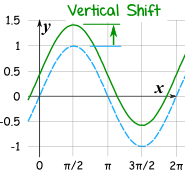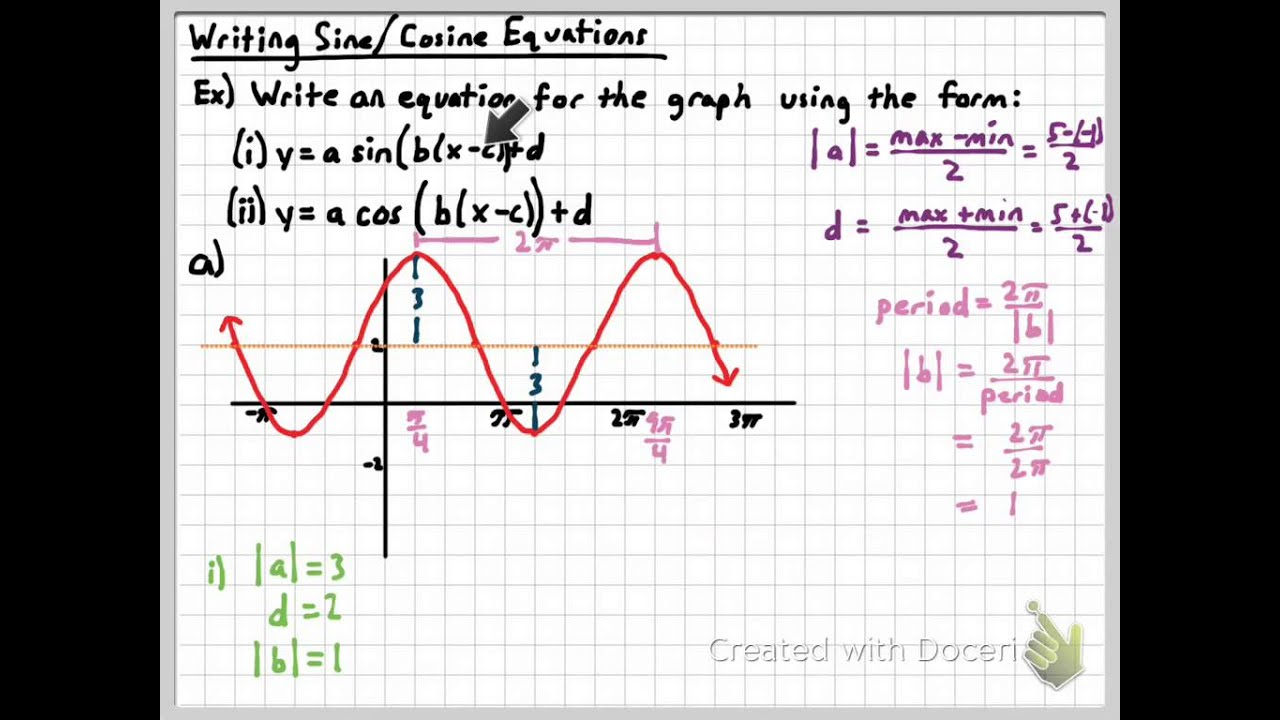# Writing Trig Eq’s

Properties of Trig Functions
About Trigonometric Functions: Trigonometric functions occur in any real world situation that continuously recurs after a certain point in time. Such real world examples could be the rise and fall between high and low tide, radio waves, electrical currents, the orbit of the earth, or even the spinning of a ferris wheel. These real world examples of sine and cosine functions are represented in a continuous graph that looks like waves. This occurs because of the recurrence of the functions periods. A period is the distance required for the function to complete one full cycle. For example, the time it takes for the highest point the tide reaches all the way to the lowest point of the tide and back to the highest point is one period. Another example is the time it takes for a ferris wheel to go around one time. Other properties are used as well to describe trigonometric functions such as the vertical shift of the function, the amplitude of the function, and phase shift of the function described below.

### About trig functions

 Sin In a right angled triangle, the sine of an angle is the length of the opposite side divided by the length of the hypotenuse. Csc In a right angled triangle, the csc of an angle is the length of the hypotenuse divided by the length of the opposite side Cos In a right angled triangle, the cos of an angle is the length of the adjacent side divided by the length of the hypotenuse. Sec In a right angled triangle, the csc of an angle is the length of the hypotenuse divided by the length of the adjacent side Tan In a right angled triangle, the tan of an angle is the length of the opposite side divided by the adjacent side. Cot In a right angled triangle, the csc of an angle is the length of the adjacent side divided by the length of the opposite side.Definitions:
Trigonometry: The branch of mathematics dealing with the relations of the sides and angles of triangles and with the relevant functions of any angles.
Sinusoidal Function: A periodic function that repeats all its values after a certain period. This is represented in a graph that oscillates.

The graph of a trigonometric function is represented by:

Y= a +(-) b SIN/COS(cX +(-) d)

a=Vertical Shift
b=Amplitude
c=2π/period
d=(phase shift)/c

+b = no reflection
-b = reflection
+d= horizontal shift left
-d= horizontal shift right

# Qualities of a Trig Functions

1. Period
The period is the distance required for the function to complete one full cycle.
Equation for period: p = 2π/c2. Amplitude
The amplitude is the value of the function to its maximum or minimum (vertically stretches the function).
Amplitude = b3. Vertical Shift
The graph moves vertically, up or down. The movement is all based on what happens to the y-value of the graph.
Vertical shift = a4. Phase Shift
Phase shift is the amount of horizontal displacement of the function from its original position.
Phase Shift = d/c###### Writing Trigonometric Functions:###### Example:###### Example Problems
1.Write an equation for the graph in the form y = A sin ( Bx + C ).

The amplitude of the graph is 2, so A = 2.

We know that one typical complete wave of the sine function starts at a y-value of 0, increases to 1, decreases through 0 and on to –1, and then increases to a y-value of 0, so the interval [-π/3, 3π +2π/3] contains one wave length of the function. The length of the interval tells us that the period is (3π +2π/3) - (-π/3) = 4π.

Using the formula: period = 2π/b , we set 4π=2π/b = and solve for B , finding that b=1/2.
We have already observed from the graph that the phase shift is -π/3, so we can use the formula:
phase shift = -c/b, set -π/3=c/(½) and solve for C , finding that c=π/6.
The equation of the graph is y=2sin((½)x+π/6)

2. Write an equation for the same graph in the form y=Acos(bx+c).

The amplitude of the graph is still 2, so A = 2.
We know that one typical complete wave of the cosine function starts at a y-value of 1, decreases through 0 to –1, and then increases through 0 to 1, so the interval [2 p /3, 4 p +2 p /3] contains one wave length of the function. The length of the interval tells us that the period is 4 p +2 p /3 - 2 p /3 = 4 p , the same period as we found in Example 1, so b=½ as before.
We can use a phase shift of 2π/3, so the formula: phase shift = -c/b becomes 2π/3=-c/(½) and c= -π/3.
The equation of the graph is also y=2cos((½)x-π/3).

Writing the Equation With Step by Step Instructions: Real World Application.

A circular Ferris wheel is 100 meters in diameter and contains several carriages. Jack and Jill enter a carriage at the bottom of the wheel and get off 20 minutes later after having gone around times 5. When a carriage is at the bottom of the wheel, it is 2 meter off the ground.Write a sinusoidal function to represent this problem.

As written in the form, y = A + B(sin/cos) (Cx + D)

1. Vertical Shift and Amplitude:
a. The best thing to find first is the maximum and minimum height of the ferris wheel. We know the ferris wheel in 100 meter in diameter, and we know it is a meter on the ground at the bottom from where you sit, so the maximum height reached is 102m and the minimum height is 2m.

b. Next the vertical shift (A) can be found. Since the vertical shift is the center of the graph, know that the ferris wheel being 100m divided by two is 50, then add 2 since the minimum point is 2 rather than zero. This gives a vertical shift of 52 for graph.

c. Now the amplitude (B) of the function can be found. Since the amplitude is the difference from the max and min to the center of the function, we can find the amplitude by subtraction 52 from 102 (the max). This gives 50 for the amplitude of the function

2. Period and Phase Shift:
a. The period would be the time it took Jack and Jill to go one time around. Since it took Jack and Jill 20 minutes to go 5 times around, we can divide the 20 minutes by 5 to get the time for one time around. This gives a period of 4. Now, to find C, we know p=2π/c, so c=2π/p (c=2π/4). When solved, C= π/2.
b. Since at zero minutes Jack and Jill were at the minimum height of the ferris wheel, no phase shift is needed.

3. Reflection and Trig Function:
a. Since our function starts at a minimum point, and we know sin starts at the midpoint and cosine at the max, it couldn't fit either of those trig functions. But, we do know reflections can be used in our function and when cosine is reflected the maximum point becomes the minimum point. In this case, our function can be a reflection of cosine, represented in our equation as a negative on the amplitude and cos after.

4. Final Equation: y = 52 - 50cos(π/2x)

Now try to write your own equal from a real world example:

On a beach high at a certain point in the water high tide occurred at midnight with a water level of 10 feet. Later, at low tide, it was 2 feet. Assuming the next high tide is exactly 12 hours later and that the height of the water is given by a sine or cosine curve, write a Sinusoidal equation to represent the rise and fall of the tide at this beach.